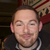+1
Completed

# Save to camera roll in a different size

``` <wire> <main> <panel name="background" height="100%" width="100%" background="#0000ee"> <panel name="contain" alias="CONTAIN" height="600" width="600" align="center"> <camera alias="CAMERA" name="panel" onclickup="picture" background="#FFFFFF" type="video" height="400" width="400" align="center" valign="top"></camera> <text name="title" text="Light" font="helvetica" size="24" width="50%" height="20%" alignment="center" valign="bottom" align="left" color="#FFFFFF" background="#000000" alpha="0.5" onclickup="light" /> <text name="title" text="Fire" font="helvetica" size="24" width="50%" height="20%" alignment="center" valign="bottom" align="right" color="#FFFFFF" background="#000000" alpha="0.5" onclickup="picture" /> </panel> </panel> </main> <actions> <action name="onstart" oninit="yes"> <assign property="var:torch" value="off"/> </action> <action name="picture"> <sync> <event type="pausePreview" target="CAMERA" wait="yes"/> <capture toalbum="test" target="CAMERA"/> <event type="resumePreview" target="CAMERA"/> </sync> </action> <action name="light"> <sync> <if lhs="[var:torch]" operator="eq" rhs="on"> <event type="torchModeOn" target="CAMERA"/> </if> <if lhs="[var:torch]" operator="eq" rhs="off"> <event type="torchModeOff" target="CAMERA"/> </if> <assign property="var:torch" value="[eval: ('[var:torch]' == 'off' ? 'on' : 'off')]" /> </sync> </action> </actions> </wire> ```